# Non Verbal Reasoning - Image Analysis

### Exercise :: Image Analysis - Section 1

6.

Find out which of the figures (1), (2), (3) and (4) can be formed from the pieces given in figure (X).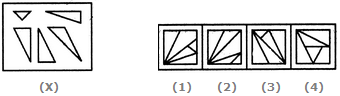A. 1 B. 2 C. 3 D. 4

Explanation:

No answer description available for this question. Let us discuss.

7.

Find out which of the figures (1), (2), (3) and (4) can be formed from the pieces given in figure (X).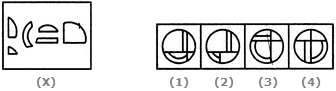A. 1 B. 2 C. 3 D. 4

Explanation:

No answer description available for this question. Let us discuss.

8.

Find out which of the figures (1), (2), (3) and (4) can be formed from the pieces given in figure (X).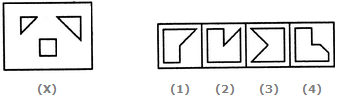A. 1 B. 2 C. 3 D. 4

Explanation: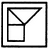9.

Find out which of the figures (1), (2), (3) and (4) can be formed from the pieces given in figure (X).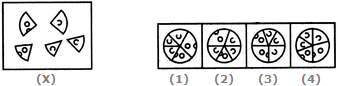A. 1 B. 2 C. 3 D. 4

Explanation:

No answer description available for this question. Let us discuss.

10.

Find out which of the figures (1), (2), (3) and (4) can be formed from the pieces given in figure (X).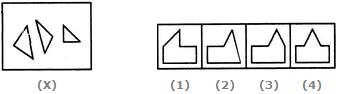A. 1 B. 2 C. 3 D. 4